Powers of Quotients MathBitsNotebook.com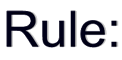For all numbers x and y and integers n and m,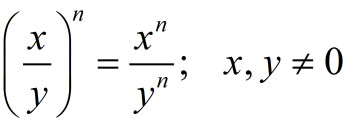BOTH the "top" and the "bottom" get raised to the new power! When a quotient is raised to a power, the numerator will be raised to the power, AND the denominator will be raised to the power. When in doubt, expand terms to see what is happening.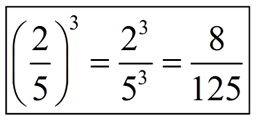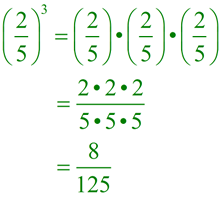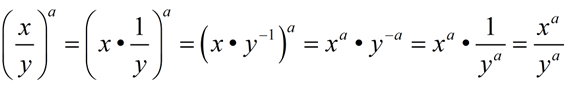Examples:

 1.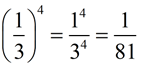Notice that the exponent is applied to both the numerator and the denominator. 2.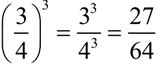3.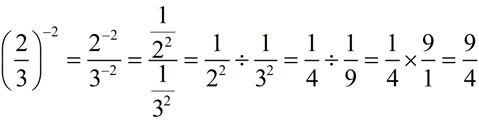Be careful on this one. Remember that the fraction bar means "divide". The negative exponent in this problem creates a division of fractions situation. Remember, when dividing fractions, invert (flip over) the second term and multiply. 4.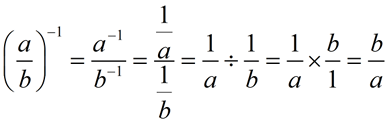This is another situation of the negative exponent creating a division of fractions situation. Did you notice in this problem that raising a fraction to a negative one power, flipped the fraction over?